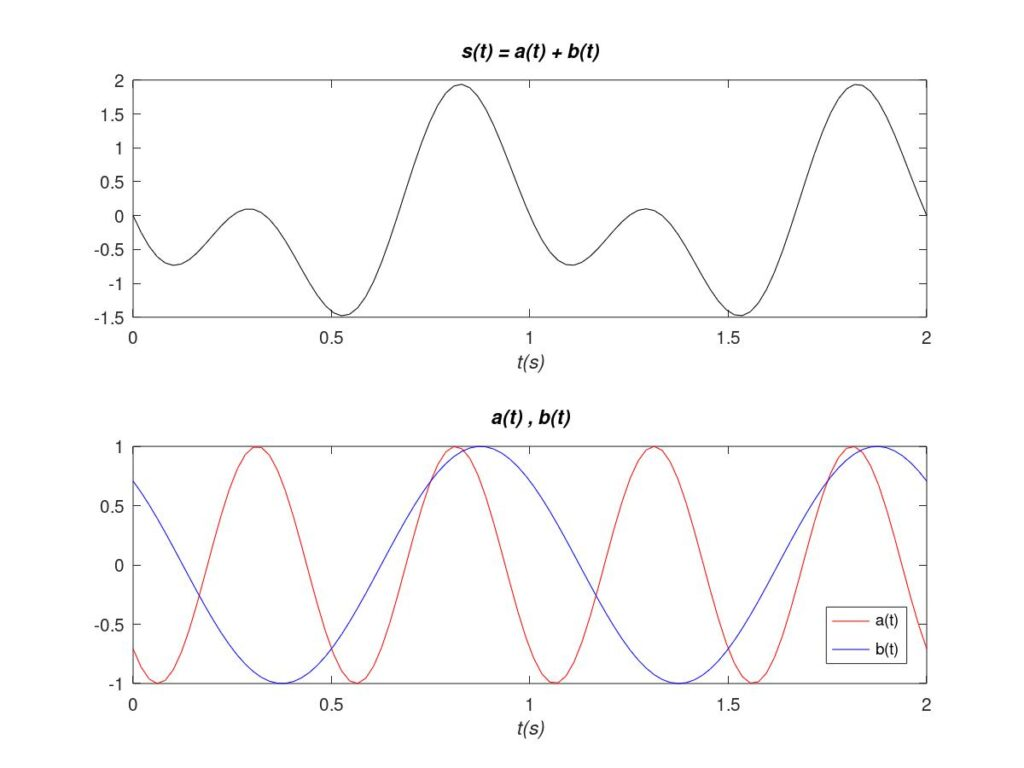# Transmission Media and Electronic Distortion

Communications are based on the sending of information by means of transmission media. This concept, the “transmission medium”, should be understood in a broad sense. The term “transmission medium” refers to the space (or electronic component) that separates any two points. Either a cable between two devices. Or a small track connecting two elements on an electronic printed circuit board. Or even the space separating two antennas thousands of kilometers apart.

In practice, at the physical level, the information sent on the transmission medium is reduced to electrical parameters (voltage, current, power, electric or magnetic field, etc.). Generically, all these parameters carrying information are called “signals”. This establishes the link that forms the theory of signal transmission media.

This section analyzes the distortion produced by the transmission media from the following perspective. First, the components that make up the signals are explained in a basic way. Next, the effects of the transmission media on these components are classified. Finally, each of these possible effects is discussed individually.

## 1. Signal Components

In general, signals can be decomposed into a sum of tones, each with its amplitude A, frequency f, and phase \varphi . As an example, the mathematical expression of a signal s(t) composed of two components, a(t) and b(t), reads as follows:

\begin{equation} s(t) = \underbrace{A_1\cos(2{\pi}f_1t +\varphi_1 ) }_{\text{a(t)}} \, + \,\underbrace{A_2\cos(2{\pi}f_2t +\varphi_2 ) }_{\text{b(t)}} \end{equation}Example of signal s(t) consisting of two tones (A_1 = 1 , f_1 = 2 , \varphi_1 = 3{\pi}/4 ; A_2 = 1 , f_2 = 1 , \varphi_2 = {\pi}/4)

## 2. Disturbances caused by Transmission Media

### 2.1 Distortion

The transmission media can produce different effects and disturbances on the signal s(t). These effects can be classified according to the parameter they directly affect: amplitude, phase, or frequencies of the individual tones that make up the signal.

On the other hand, a signal is said to have suffered distortion in the transmission medium when its “appearance” changes with respect to the original signal. This phenomenon occurs when the effects on amplitude and/or phase and/or frequency of the individual tones are not uniform. On the contrary, a transmitted and undistorted signal maintains the same “aspect” as the original signal. In the latter case, the amplitude and/or phase and/or frequency effects of the individual tones are uniform.

### 2.2 Distortion Classification

Next, electrical distortion is classified according to the parameters affected by the transmission medium or component:

• Frequencies composing the signal: When the medium is linear, the frequencies obtained at the output are already present at the input. On the contrary, a non-linear medium generates additional frequencies in the output signal, ensuring distortion.
• Amplitude: If a linear transmission produces the same gain/attenuation of the amplitude at all frequencies, the resulting signal can maintain its original appearance. Conversely, the signal will be distorted if there are at least two frequency components that are subjected to different gain/attenuation.
• Phase: When in a linear transmission the phase of all tones varies linearly with frequency, all components suffer the same time delay. Consequently, assuming that the amplitude of the tones does not change, the overall signal does not suffer distortion because it is a delayed replica of the original signal. However, if some frequencies are delayed with respect to others, distortion is ensured.

Note that this classification is not rigid. A transmission medium, or a component, can produce different combinations of the effects presented.

In addition, it should be kept in mind that rarely is a medium totally innocuous with respect to any parameter. In general, when speaking of distortion-free cases, reference is made to situations where the distortion is so small that it can be neglected.

Finally, it should be noted that noise, in practice, is always present in one form or another in any component or transmission. Noise always generates distortion, although, if it is low enough, it can also be neglected.

## 3. Summary Table and Examples

As a summary, the following table summarizes the effects of the transmission media on the frequencies that make up a signal, and on the amplitudes and phases of these frequencies:

Note that in this article distortion has been explained from the frequency point of view. By changing the point of view, the nomenclature may vary between different texts. As an example, in this summary we call amplitude distortion the case in which the frequencies that make up a signal change their amplitude in a non-uniform way. Sometimes, in other texts, this case is called frequency distortion.

Each of the disturbances shown in the table is explained individually below with simple examples. For a more formal analysis of the linear distortion, please refer to this link.

Bibliography
 Communication Systems, A. Bruce Carlson.

Subscription
If you liked this contribution, feel free to subscribe to our newsletter: